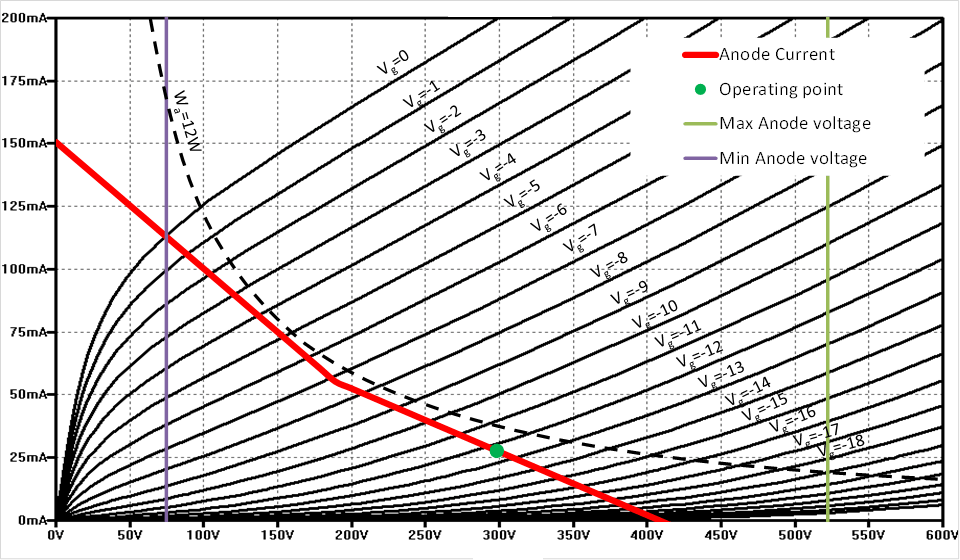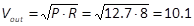We adopt a push-pull configuration in ultra-linear mode. Ultra-linear mode and push-pull configurations were introduced respectively in Section 2.2.4 and Section 4.1.4. The overall schema of the power stage, including values of all components, is given in Figure 48. Let us see how these values were chosen.

EL84 datasheet specifies that anode and screen voltage can be set to 300V and max anode power dissipation is 12 Watts.

Figure 49 shows the anode characteristic graph of an EL84 vacuum tube, when anode voltage is 300V and ultra-linear configuration is obtained feeding the screen with 40% of the primary turns.

An output transformer for push-pull operation, with reactive load of 8K Ohm, provides each vacuum tube with a load of 4K Ohm, when operating in Class A. By setting the anode bias current to 28mA, we obtain the loadline depicted by the red line, which stays below the 12 Watts max allowed anode power dissipation.

From Figure 49 we can see that the grid voltage bias, needed to obtain an anode bias current of 28mA, is around -12V.

The amplifier operates in Class AB. More specifically, it operates in Class A when the anode voltage is in the range from 190V to 410V. When out of this range, as explained in Section 4.1.6, one of the two vacuum tubes quits conducting and the other one sees just one fourth of the whole transformer impedance. In fact, below 190V the reactive load seen by the conducting vacuum tube is 2K Ohm and the slope of the load line increases accordingly.

With this configuration, the maximum allowed peak amplitude of the input signal is 12V. In fact, when the input voltage is 12V, since the input signal is added to the grid voltage bias, the grid voltage becomes zero. Under these conditions, the vacuum tube saturates, since it has maximum conduction, and anode voltage is minimum.Figure 49: Average anode characteristic graph and loadline of EL84 in ultra-linear configuration.The figure shows the anode characteristic graph of an EL84 vacuum tube operating in ultra-linear configuration. Anode voltage is set to 300V and screen is fed with 40% of primary turns. Red line represents the push-pull loadline with an 8K Ohm push-pull transformer and bias current set to 28mA.

The grid stopper resistor Rg, generally used with an EL84 vacuum tube, is 1K Ohm. We also use a 1K Ohm screen stopper resistor Rscreen to connect the EL84 screens to the ultra-linear taps of the transformer. EL84 datasheet specifies that maximum grid to cathode resistance, with fixed bias, is 300K Ohm. Accordingly, we can use a grid leak resistor Rl of 200K Ohm to give some margin to the resistance of the bias voltage circuit.

Using the calculation given in Example 6 we can determine the value of the inter-stage coupling capacitor Cs, between the phase splitter and the output stage. To obtain a high pass filter at 7Hz, with a grid leak resistor of 200K Ohm, we set Cc to 0.1μF.

The decoupling capacitor Cd, to prevent residual signal at the grid leak resistor to go to the other vacuum tubes when fixed bias is used, can be computed according to Example 3. Suppose that we want to short to ground all residual signals with a frequency above 2 Hz, and that the only resistance seen by the capacitor is the 200K Ohm grid leak itself. In this case, a Cd=0.47μF accomplishes to this task. Note also that, since the grid bias voltage is provided using a voltage divider, as discussed in Section 5.3, part of the residual signal at the grid leak resistor is also shorted to ground by this voltage divider further reducing the cut-off frequency of the low pass filter.

### 6.1.1    Maximum output power estimation and voltage gain of the power stage

A precise estimation of the maximum output power of the power stage is difficult. It is affected by the non-linearity of the vacuum tubes and the impedance variation of the output transformer in class AB operation. However, a quite accurate estimation can be obtained as follows. From the loadline drawn on the average anode characteristic graph in Figure 49 we can see that when the inputs signal goes from 0 to 12V, the anode voltage of one vacuum tube goes from the 300V quiescent condition to 75V. The peak of the voltage variation at the anode of the vacuum tube is 300V-75V=225V. In a push-pull amplifier, when one tube is at the highest peak, the other is at the lowest peak. When the anode voltages of the two vacuum tubes are given to the two ends of the output transformer, the peak of the voltage variation is therefore doubled, and the peak voltage seen by the transformer is 2∙225V=450V. This expressed in RMS gives 450V*0.707=318.15V. At this point, knowing the reactive impedance of the transformer, we are able to estimate the expected maximum RMS power seen at the primary of the transformer as P=V2/R=318.152/8K=12.7 Watts RMS. In an ideal output transformer, this power is entirely transferred to the output and represents the maximum RMS output power.

With this, we are also able to estimate the voltage gain after the output transformer. Suppose that we use 8 Ohm speakers. Given that the maximum RMS output power is 12.7 Watts, the maximum output voltage with an 8 Ohm load isVolts RMS.

The maximum RMS input voltage that can be applied to the power stage is Vin=12V∙0.707=8.48V. Therefore, the voltage gain of the power stage, after the output transformer, is A=Vout/Vin= 10.1/8.48=1.19. Expressed in dB, we have Adb=20∙log(1.19)=1,51 dB. We will see that the value of the voltage gain will be useful when designing the global feedback circuit.